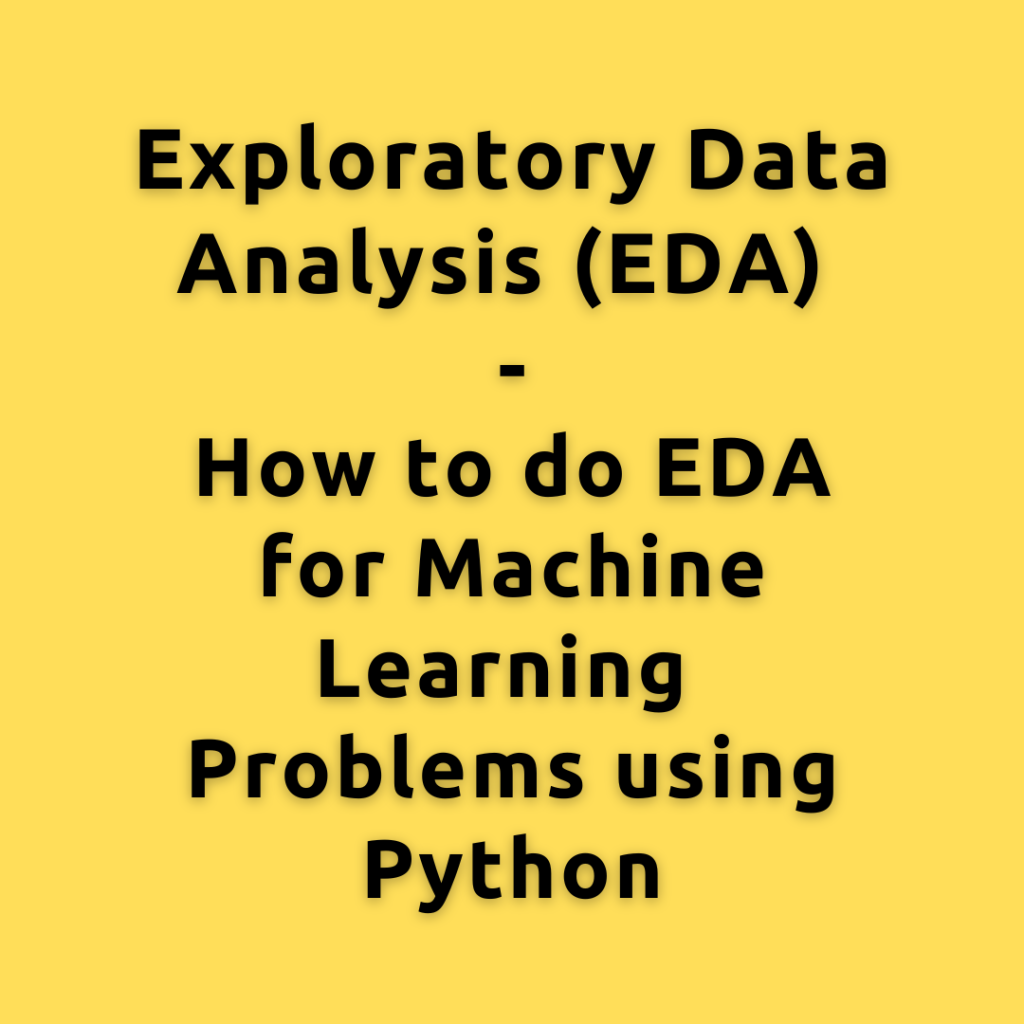## Exploratory Data Analysis (EDA) – How to do EDA for Machine Learning Problems using Python

Exploratory Data Analysis, simply referred to as EDA, is the step where you understand the data in detail. You understand each variable individually by calculating frequency counts, visualizing the distributions, etc. Also the relationships between the various combinations of the predictor and response variables by creating scatterplots, correlations, etc. EDA is typically part of every …